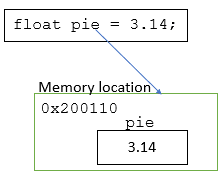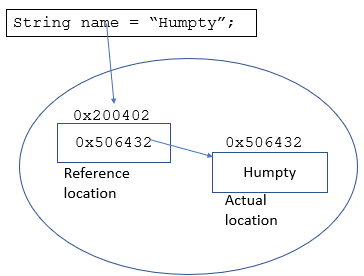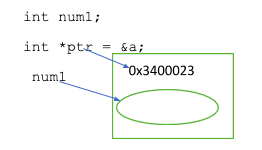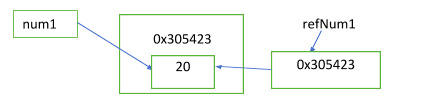Need a discount on popular programming courses? Find them here. View offers

Don't have an account?

Have you read our submission guidelines?

Go back to

# Pass by Reference

There are three ways to pass variables to a function – pass by value, pass by pointer and pass by reference. The most common language that uses pass by reference in C++. To discuss pass by reference in detail, I would like to explain to you the other two ways as well, so that the concepts are planted in your mind forever. The examples I have written are in C++ because C++ uses all the three and it will be easier for us to compare and understand each of them. If you would like to learn more about C++, do it here.

## Pass by value

When you call a function with pass by value, two copies of variables with the same value are created. In effect, whatever changes are made to the variables inside the called function are not reflected to the actual variables with which the function is called (because they are two different copies whose memory addresses are different). For example –

```void doubleTheValue(int a)
{
a = a*2;
}
int main()
{
int a = 5;
cout << "Value of a before calling double function = " << a << "\n";
doubleTheValue(a);
cout << "Final Value of a = " << a << "\n";
}
```

You might think that count will print the value as 10. But that is not the case. The value of a in the main function will remain 5 even after calling the doubleTheValue function.

## Pass by reference

Why was the value not getting changed in the previous example? Because, there were two values pointing to different addresses in the memory! Passing by reference helps solve this issue by passing the memory location of the variable to the called function. That means, both the passed variable and the parameter used in the called function point to the same location and are always in sync – any change to the parameter reflects in the variable as well. Kudos! Let us change the above example –

```void doubleTheValue(int &param)
{
param = param*2;
}
int main()
{
int a = 5;
cout << "Value of a before calling double function = " << a << "\n";
doubleTheValue(a);
cout << "Value of a = " << a << "\n";
}
```

&a points to the memory location of a. When the function executes, it picks the value of a from that memory location and changes it. This time, cout gives us ‘10’ as the answer.

With this basic overview, let us now move on to some more details of pass by reference.

### How Java and other languages ‘pass by reference’

While most of the newer languages deal with pass by reference with a different name, the underlying concept remains the same. For example, Java passes objects (class types) by reference, but the primitives like int, double etc are passed by value. Consider –

```public class Student {
int rollNo;
char gender;
boolean pass;
}
```

Let us say a function changeDetails(Student stud1) passes the object of Student. This object has a memory location and any changes to the Student object will be reflected in the address. But what about the primitive types?

Their values are also changed even though they are pass by values, because they reside inside the object and are accessed through the same memory location. So, when we do this will create a copy of the variable and the value in the stud1 object will not be changed!

Same way, if you pass individual primitive variables, the original value will not be changed. However, if you pass the whole object, the values are changed. The languages like Python, JavaScript, Ruby and PHP do the same!

This entire discussion brings us to an important point – what is the difference between value types and reference types?

### Value types vs reference types

A quick comparison will help us understand the differences and usage clearly –

 VALUE TYPE REFERENCE TYPE All primitive data types are value types. For example – int, double, byte, char, long, short, etc… Reference data types can be String, arrays, class, delegates The value is stored in memory and can be directly accessed. Reference type stores the memory location that contains the data. The memory location acts as a pointer to the actual memory location.Now that you are able to picturise the difference between pass by value and pass by reference, let us understand one more concept – pass by pointer.

## Pass by pointer

In our previous example of doubleTheValue function, we are passing the value of ‘a’ by reference using ‘&’. We can do the same thing by passing the value through a pointer. Let us modify our doubleTheValue example and include a pointer –

```void doubleTheValue(int *ptr)
{
int a = *ptr;
*ptr = a*2;
cout << "Address of a (ptr) = " << ptr << "\n";
cout << "Initial value of a = " << a << "\n";
}
int main()
{
int a = 5;
doubleTheValue(&a);
cout << "Final Value of a = " << a << "\n";
}
```

As we are sending the address of a, which is ptr or pointer to the location, value of a is accessed as *ptr. This will give the output as –

```Address of a (ptr) = 0x7ffc0f8fa3ec
Initial value of a = 5
Final Value of a = 10
```

The output is the same whether we do pass by pointer or pass by reference. In both cases, we are referring to the memory location where the variable ‘a’ is stored and modify the value directly from the single address. There is no other copy of a created, unlike in pass by value.

So, what is the difference between pass by pointer and pass by reference?

Before doing a tabular comparison, I want to show you another example, where we can easily picturize the differences.

#### Quick overview of pointer

A pointer can store memory address of any variable (and not value), named or unnamed. It can also point to null or empty. For example –Same way, we can say – int *ptr2 = new int;

where there is no variable assigned to the new int, but a memory location has been created along with a pointer ptr2.

You can also assign a pointer to null!

#### Quick recap of reference

On the other hand, reference is an alias for a variable. Let us say,

```int num1 = 20;
int &refNum1 = num1;
```

Both num1 and refNum1 point to the same location now.Because of this, whenever we change the value of num1, refNum1 will change and vice versa. Basically, we are changing the value at the memory location, which has two names – num1 and refNum1.

We see that the reference variable and pointer both hold the address of a variable, however reference value should always be initialized. They cannot hold empty or null values.

int &refNum1; //will give you compilation error

### Pointer vs Reference

Here is a sample code where we will use pointers and references. Read it step by step –

```#include
using namespace std;
int main() {
int maths = 99, science = 70;
// Create a pointer pointing to maths
int * pMaths = &maths; // Explicit referencing
// value of variable that the pointer is pointing to
cout << *pMaths << "\n";
// Both give the memory address of maths variable
cout << &maths << "\n";
cout << pMaths << "\n";
// Gives location of pointer
cout << &pMaths << "\n";
// pointer can be reassigned to point to some other address
pMaths = &science; // pMaths no longer points to maths
cout << pMaths << "\n"; // prints the address of science
cout << &pMaths << "\n"; // pointer location is same though points to different variable
// Create a reference (alias) to maths
int & refMaths = maths; // maths and not &maths
// not pointer *refMaths, just refMaths (value of maths)
refMaths = 92;
cout << refMaths << "\n"; // 92
cout << maths << "\n"; //92
//Both &maths and &refMaths give memory address of maths variable</span
cout << &maths << "\n";
cout << &refMaths << "\n";
//This code is wrong, you cannot assign reference to another. In other words "invalid conversion from 'int*' to 'int'"
//refMaths = &science;
// This will change maths and refMaths both to the value of science
refMaths = science;
science++;
cout << refMaths << "\n"; // 70
cout << maths << "\n"; // 70
cout << science << "\n"; // 71
}
```

The code is modified version of the original code from here.

### Pass by Pointer vs Pass by Reference: Head to Head Comparison

Now, let us compare the two operations side-by-side –

 PASSING VARIABLES BY POINTER PASSING VARIABLES BY REFERENCE Creates a pointer that stores memory address of a variable Creates another name for the same variable (alias) The dereferencing operator * gives the value of the variable Value can be implicitly referenced using the reference name. Can point to nothing, null or empty. Has to be initialized during declaration Can be reassigned to another memory location Can’t be reassigned to another memory address Uses ‘&’ to reference the address of variable. For example – int * pMaths = &maths; Also, cout << &pMaths << "\n"; gives the address of the pointer Using &, we can get the address of reference (because the address is the same as that of the original variable)

## Final word

Hope the examples and comparisons have given you enough clarity on pass by reference and how it is different from pass by value and pass by pointer. Though pass by reference and pass by pointers serve the same purpose, there are subtle differences between both. Remember that pointer is a reference, but the vice-versa may not be true. As we have seen, most objects use reference because it is quicker and does not have to deal with the additional features that pointer gives. When you have to reassign a location, use pointer. Otherwise, always prefer references!

Tutorials you Might be Interested In:

STAY IN LOOP TO BE AT THE TOP

#### Welcome to the club and Thank you for subscribing!

By Ramya Shankar

A cheerful, full of life and vibrant person, I hold a lot of dreams that I want to fulfill on my own. My passion for writing started with small diary entries and travel blogs, after which I have moved on to writing well-researched technical content. I find it fascinating to blend thoughts and research and shape them into something beautiful through my writing.

View all post by the author

Disclosure: Hackr.io is supported by its audience. When you purchase through links on our site, we may earn an affiliate commission.